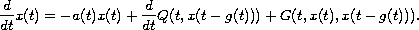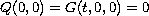Electron. J. Diff. Eqns., Vol. 2005(2005), No. 142, pp. 1-11.

### Periodicity and stability in neutral nonlinear differential equations with functional delay Youssef M. Dib, Mariette R. Maroun, Youssef N. Raffoul

Abstract:
We study the existence and uniqueness of periodic solutions and the stability of the zero solution of the nonlinear neutral differential equationIn the process we use integrating factors and convert the given neutral differential equation into an equivalent integral equation. Then we construct appropriate mappings and employ Krasnoselskii's fixed point theorem to show the existence of a periodic solution of this neutral differential equation. We also use the contraction mapping principle to show the existence of a unique periodic solution and the asymptotic stability of the zero solution provided that.

Submitted November 30, 2004. Published December 6, 2005.
Math Subject Classifications: 34K20, 45J05, 45D05.
Key Words: Krasnoselskii; contraction; neutral differential equation; integral equation; periodic solution; asymptotic stability

Show me the PDF file (241K), TEX file, and other files for this article.

 Youssef M. Dib Department of Mathematics, University of Louisiana Lafayette, LA 70504-1010, USA email: youssef@louisiana.edu Mariette R. Maroun Department of Mathematics, Baylor University Waco, TX 76798-7328, USA email: Mariette_Maroun@baylor.edu Youssef N. Raffoul Department of Mathematics, University of Dayton Dayton, OH 45469-2316, USA e-mail: youssef.raffoul@notes.udayton.edu